# High School Math : Understanding Sine, Cosine, and Tangent

## Example Questions

### Example Question #1 : Understanding Sine, Cosine, And Tangent

If the polar coordinates of a point are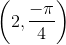, then what are its rectangular coordinates?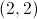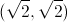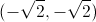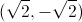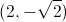Explanation:

The polar coordinates of a point are given as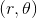, where r represents the distance from the point to the origin, and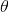represents the angle of rotation. (A negative angle of rotation denotes a clockwise rotation, while a positive angle denotes a counterclockwise rotation.)

The following formulas are used for conversion from polar coordinates to rectangular (x, y) coordinates.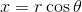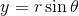In this problem, the polar coordinates of the point are, which means that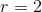and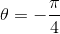. We can apply the conversion formulas to find the values of x and y.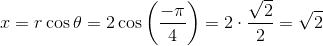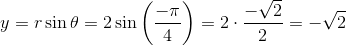The rectangular coordinates are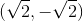.

The answer is.

### Example Question #1 : Trigonometry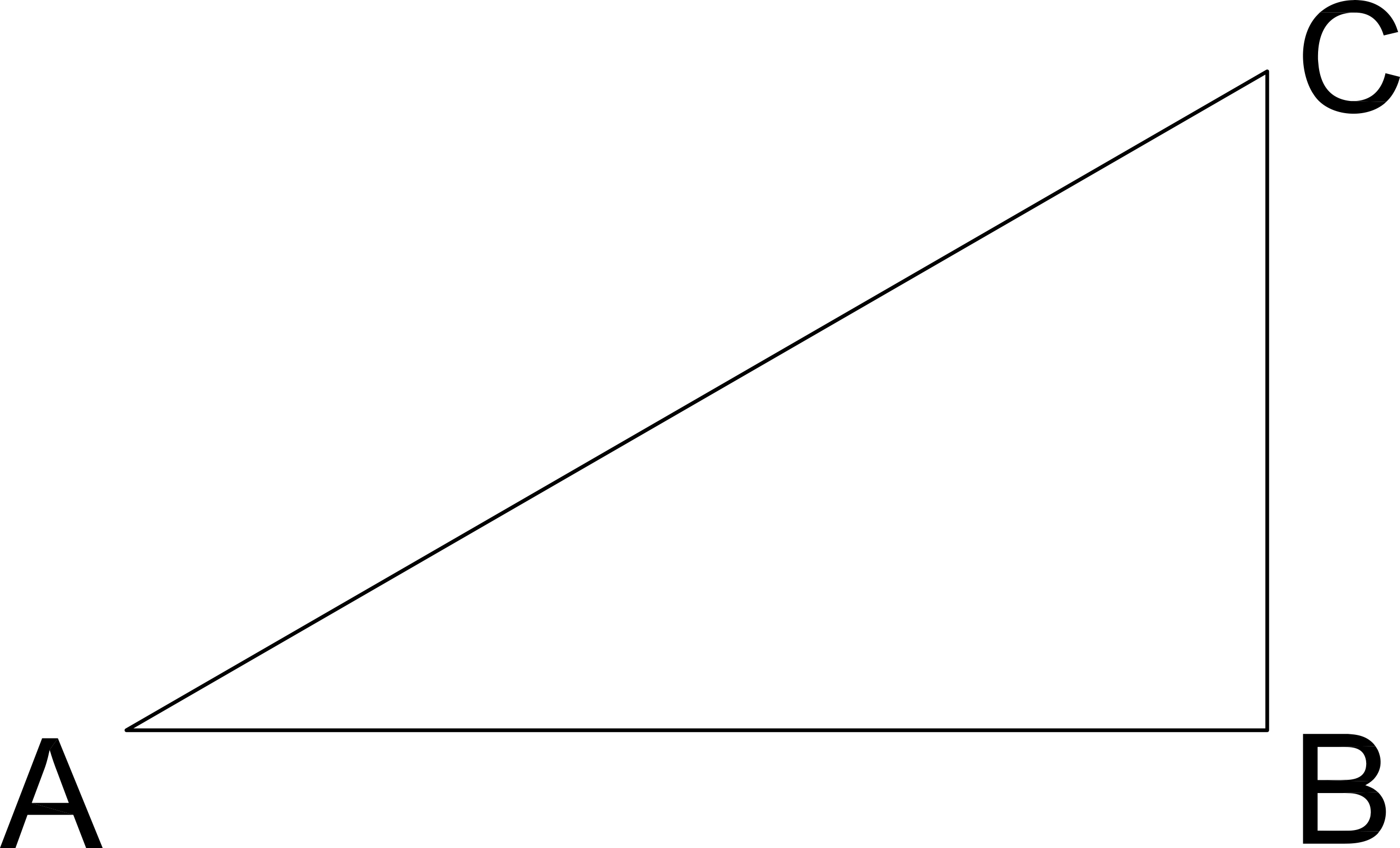What is the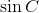?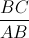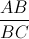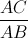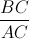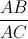Explanation: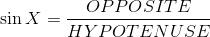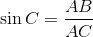### Example Question #3 : Understanding Sine, Cosine, And Tangent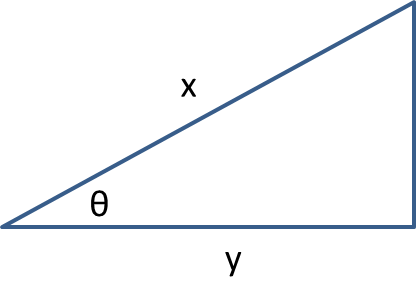In the right triangle above, which of the following expressions gives the length of y?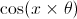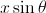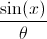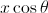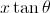Explanation: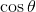is defined as the ratio of the adjacent side to the hypotenuse, or in this case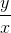. Solving for y gives the correct expression.

### Example Question #4 : Understanding Sine, Cosine, And Tangent

What is the cosine of?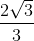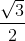Explanation:

The pattern for the side of a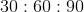triangle is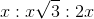.

Since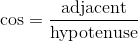, we can plug in our given values.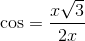Notice that the's cancel out.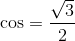### Example Question #1 : How To Find Negative Sine

If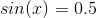, what is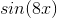ifis betweenand?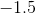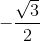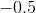Recall that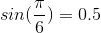.
Therefore, we are looking for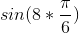or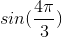.
Now, this has a reference angle of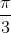, but it is in the third quadrant. This means that the value will be negative. The value of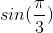is. However, given the quadrant of our angle, it will be.